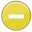#eccentricities 中文解釋 wordnet sense Collocation Usage Collins Definition
Noun
/ˌeksenˈtrisitē/,Font size:eccentricities, plural;
1. The quality of being eccentric

2. An eccentric act, habit, or thing
• - her eccentricities were amusing rather than irritating
3. Deviation of a curve or orbit from circularity

4. A measure of the extent of such deviation
• - Halley's Comet has an eccentricity of about 0.9675

1. (eccentricity) strange and unconventional behavior
2. (eccentricity) (geometry) a ratio describing the shape of a conic section; the ratio of the distance between the foci to the length of the major axis; "a circle is an ellipse with zero eccentricity"
3. (eccentricity) a circularity that has a different center or deviates from a circular path
4. (Eccentricity (behaviour)) In popular usage, eccentricity refers to unusual or odd behavior and "socially awkward" on the part of an individual. This behavior would typically be perceived as unusual or unnecessary, without being demonstrably maladaptive. ...
5. (Eccentricity (graph theory)) In the mathematical field of graph theory, the distance between two vertices in a graph is the number of edges in a shortest path connecting them. This is also known as the geodesic distance
6. (Eccentricity (mathematics)) In mathematics, the eccentricity, denoted e or , is a parameter associated with every conic section. It can be thought of as a measure of how much the conic section deviates from being circular.
7. (Eccentricity (mechanics)) In mechanical engineering, an eccentric is a circular disk (eccentric sheave) solidly fixed to a rotating axle with its centre offset from that of the axle (hence the word "eccentric", out of the centre).
8. (Eccentricity (orbit)) The orbital eccentricity of an astronomical body is the amount by which its orbit deviates from a perfect circle, where 0 is perfectly circular, and 1.0 is a parabola, and no longer a closed orbit.
9. (Eccentricity (Maximum)) In High Intensity Discharge lamps, the Bulb to Arc Angle is the angle off of center between electrodes and bulb. The Bulb to Base Angle is the angle off of center that the bulb is from the base.
10. (Eccentricity) Like concentricity, a measure of the center of a conductor's location with respect to the circular cross section of the insulation. Expressed as a percentage of displacement of one circle within the other.
11. (Eccentricity) The extent to which the Earth's orbit around the Sun departs from a perfect circle.
12. (eccentricity) the condition resulting from the inside and outside diameters not having a common center. See eccentric wall.
13. (ECCENTRICITY) the variations of the shaft surface in reference to the centerline of the shaft.
14. (Eccentricity) The deviation of an ellipse from a perfect circular shape.
15. (Eccentricity) The out-of-roundness of a roll, core, or mandrel. Eccentricity usually is expressed as TIR (total indicated runout) in mils. When eccentricity is present, points on the roll surface do not rotate in the same axial circle.
16. (Eccentricity) is the rate or amount of flattening of an aspheric curve. The higher the eccentricity, the more quickly the lens flattens from the center to periphery.
17. (ECCENTRICITY) Degree to which the ellipse of an elliptical orbit is flattened.
18. (Eccentricity) (1) originality without sense. (2) a method of distinction so cheap that fools employ it to accentuate their incapacity.
19. (Eccentricity) Deviation from a common center, for example, the inner and outer walls of a round tube. The difference between the mean wall thickness and minimum or maximum wall thickness at any one cross section. ...
20. (Eccentricity) Variation in radius of an information track from the true axis of rotation of the disc. May be confused with runout.
21. (Eccentricity) an oddity or peculiarity, as of conduct, an interesting man, known for his eccentricities.
22. (Eccentricity) in geometry, a property of a conic section (circle, ellipse, parabola, or hyperbola).
23. eccentricity (symbol e) - a measure of a conical orbit shape; it shows how much this deviates from a circle. If e = 0, the orbit is a circle. If e < 1, the orbit is an ellipse, if e > 1 it is a hyperbola, and if e = 1 it is a parabola. ...
24. (n.): oddities; unconventionalities